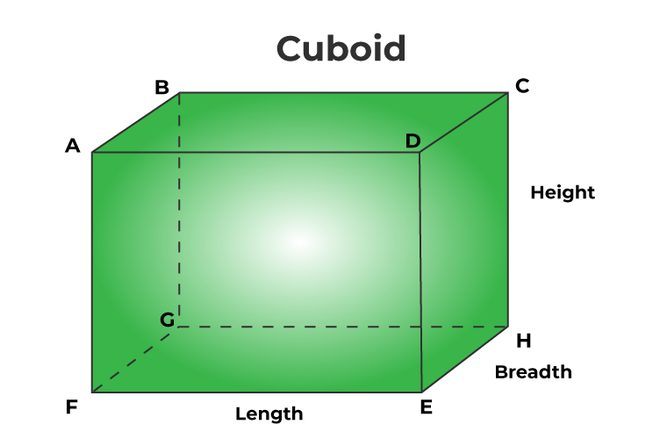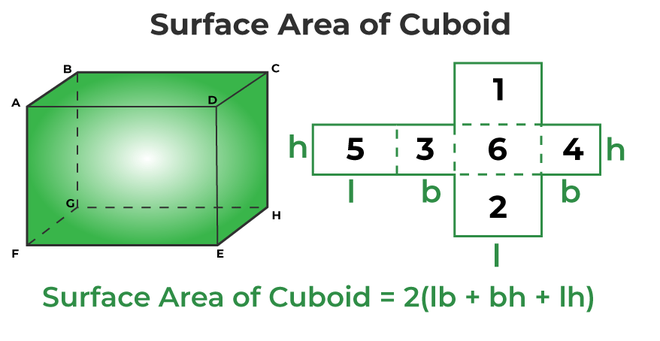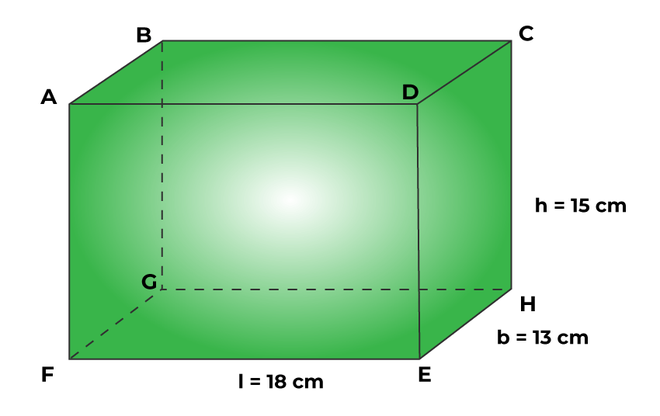Open in App
Not now

# Surface Area of Cuboid

• Last Updated : 06 Sep, 2022

The surface area of a cuboid is the total space occupied by all its surfaces/sides. In geometry, a three-dimensional shape having six rectangular faces is called a cuboid. A cuboid is also known as a regular hexahedron and has six rectangular faces, eight vertices, and twelve edges with congruent, opposite faces. It is a three-dimensional form of a rectangle with four lateral faces and two faces at the top and bottom. Some examples of cuboids that we regularly see are bricks, geometric boxes, shoe boxes, packaging boxes, etc. Let’s learn in detail about the surface area of a cuboid, along with examples.

## What is the Surface Area of a Cuboid?

The surface area of a cuboid is the total area covered by all of its surfaces, and since the cuboid is the 3-D form of a rectangle, therefore, along with length and breadth, the height of the cuboid is also involved in finding the surface area. Surface area and volume are calculated in 3-D figures. The surface area of a cuboid is measured in square units (For example, cm2, m2, etc).## Surface Area of a Cuboid Formula

A cuboid has 2 kinds of surface area. The surface area of a cuboid is calculated based on what type of surface area is required. The formulas for both types of surface areas are different. They are:

1. Total surface area of a cuboid
2. Lateral surface area of a cuboid

The total surface area of a cuboid is the area of all 6 sides of the cuboid. However, the lateral surface area of a cuboid is the area of only 4 sides (base and top excluded). Imagine there is a room in the shape of a cuboid; if only the walls of the room (excluding the ceiling) require painting, then the lateral surface area of the room will be taken into account for the paint used, and the total surface area of the room is the sum of the 4 walls + ceiling + floor. Both the total surface area and lateral surface area of a cuboid are calculated in terms of length (l), breadth(b), and height(h). The formulas are:

Lateral surface area of a cuboid = 2h(l + b) square units

Total surface area of a cuboid = 2 (lb + bh + lh) square units

Note: If the surface area is asked without giving any information or specification, then the total surface area of the cuboid is calculated.

## Derivation of Surface Area of a Cuboid

The lateral and total surface areas of a cuboid are the two types of surface areas of a cuboid. The lateral surface area of a cuboid is the area occupied by its lateral faces, whereas the total surface area of a cuboid is the area occupied by all its six rectangular faces.

### Total Surface Area of Cuboid

The total surface area of a cuboid can be calculated by first calculating the area of all the sides and then adding all six sides. Let us consider that “l” is the length of the cuboid, “b” is the breadth of the cuboid, and “h” is the height of the cuboid.We know that in a cuboid, opposite faces are equal, i.e., ABCD = PQRS, APSD = BQRC, and ABQP = DCRS. Now, the total surface area of the cuboid is equal to the sum of the areas of its six rectangular faces. The total surface area of the cuboid = Area of [ABCD + APSD + ABQP + PQRS + BQRS + DCRS].

Area of [ABCD +APSD + ABQP + ABCD +APSD + ABQP] = 2 Area of [ABCD +APSD + ABQP]

• The area of the rectangle ABCD = l × h
• The area of the rectangle APSD = b × h
• The area of the rectangle ABQP = l × b

Therefore,

Total surface area of the cuboid (TSA) = 2 (lb + bh + lh) square units

### Lateral Surface Area of a Cuboid

The lateral surface area of a cuboid is the area occupied by its lateral faces. Hence, the lateral surface area of the cuboid = The total surface area of the cuboid – The area of the top and bottom faces of the cuboid

= 2 (lb + bh + lh) – Area of [ABPQ + CDRS}

= 2 (lb + bh + lh) – 2 Area of (ABPQ)

= 2 (lb + bh + lh) – 2lb

= 2 (bh + lh) = 2h(l + b)

Hence,

Lateral surface area of a cuboid (LSA) = 2h(l + b) square units

## How to Find the Surface Area of Cuboid?

The surface area of a cuboid is the total area of all the sides/surfaces of the cuboid. In order to understand how to find the surface area of a cuboid, let’s take a look at the given steps:

Step 1: Observe and note down the dimensions of each size. In case the dimensions have different units, convert them into one unit.

Step 2: Use the formula for the total surface area of the cuboid: that is, TSA = 2 (lb + bh + lh) square units

Step 3: Note down the area contained in proper units.

Let’s consider an example to determine the surface area of the cuboid using its formula.

Example: Calculate the total surface area of a cuboid if its length, breadth, and height are 8 cm, 3 cm, and 5 cm, respectively.

Solution:

Step 1: Note down the dimensions of the given sphere. Here, the length, breadth, and height are 8 cm, 3 cm, and 5 cm.

Step 2: We know that the total surface area of a cuboid = 2 (lb + bh + lh). So, substitute the value of the given dimensions in the equation = 2 [(8 × 3) + (3 × 5) + (8 × 5)] = 158 sq. cm.

Step 3: Hence, the total surface area of a cuboid is 158 sq. cm.

## Solved Examples on Surface Area of a Cuboid

Example 1: Determine the lateral surface area of a cuboid if its length, breadth, and height are 15 in, 8 in, and 12 in, respectively.

Solution:

Given data,

The length of a cuboid (l) = 15 in

The breadth of a cuboid (b) = 8 in

The height of a cuboid (h) = 12 in

We have,

Lateral surface area of a cuboid = 2h(l + b)

= 2 × 12 (15 + 8)

= 24 × 23 = 552 square inches.

Hence, the lateral surface area of the given cuboid is 552 square inches.

Example 2: What is the total surface area of a cuboid shown in the figure below:Solution:

Given data,

The length of a cuboid (l) = 18 cm

The breadth of a cuboid (b) = 13 cm

The height of a cuboid (h) = 15 cm

We have,

Total surface area of a cuboid = 2 (lb + bh + lh)

= 2 [(18 × 13) + (13 × 15) + (18 × 15)]

= 2 [234 + 195 + 270]

= 2  = 1398 cm2

Hence, the total surface area of the given cuboid is 1398 cm2.

Example 3: Calculate the height of the cuboid whose lateral surface area is 360 square units and whose length and breadth are 12 units and 8 units, respectively.

Solution:

Given data,

The length of a cuboid (l) = 12 units

The breadth of a cuboid (b) = 8 units

The lateral surface area = 360 square units

We have,

Lateral surface area of a cuboid = 2h(l + b)

⇒ 2h (12 + 8) = 360

⇒ h × (20) = 360/2 = 180

⇒ 20h = 180⇒ h = 180/20

⇒ h = 9 units

Hence, the height of the given cuboid is 9 units.

Example 4: Determine the length and the total surface area of a cuboid whose lateral surface area is 960 sq. in and whose breadth and height are 12 in and 16 in, respectively.

Solution:

Given data,

The breadth of a cuboid (b) = 12 in

The height of a cuboid (h) = 16 in

The lateral surface area = 960 square inches

We know that,

Lateral surface area of a cuboid = 2h(l + b)

⇒ 2 × 16 (l + 12) = 960

⇒ 32 (l + 12) = 960

⇒ (l + 12) = 960/32 = 30

⇒ l = 30 – 12 = 18 in

We have,

Total surface area of a cuboid = 2 (lb + bh + lh)

= 2 [(18 × 12) + (12 × 16) + (18 × 16)]

= 2 [ 216 + 192 + 288]

= 2 ×  = 1398 square inches

Hence, the length and the total surface area of the cuboid are 18 in and 1398 sq. in, respectively.

Example 5: Calculate the total surface area of a cuboid if its length, breadth, and height are 10 in, 5 in, and 8 in, respectively.

Solution:

Given data,

The length of a cuboid (l) = 10 in

The breadth of a cuboid (b) = 5 in

The height of a cuboid (h) = 8 in

We have,

Total surface area of a cuboid = 2 (lb + bh + lh)

= 2 [(10 × 5) + (5 × 8) + (10 × 8)]

= 2 × [50 + 40 + 80]

= 2 × (170) = 340 sq. in

Hence, the total surface area of the given cuboid is 340 sq. in.

Example 6: Determine the lateral and total surface areas of a cuboid whose length, breadth, and height are 21 cm, 16 cm, and 18 cm, respectively.

Solution:

Given data,

The length of a cuboid (l) = 21 cm

The breadth of a cuboid (b) = 16 cm

The height of a cuboid (h) = 18 cm

We have,

Lateral surface area of a cuboid = 2h(l + b)

= 2 × 18 (21 + 16)

= 36 × 37 = 1332 sq. cm

Total surface area of a cuboid = 2 (lb + bh + lh)

= 2 [(21 × 16) + (16 × 18) + (21 × 18)]

= 2 [336 + 288+ 378] = 2 × 1002

= 2004 sq. cm

Therefore, the lateral surface area and the total surface area of a cuboid are 1332 sq. cm and 2004 sq. cm, respectively.

## FAQs on Surface Area of a Cuboid

Question 1: What is the difference between the total surface area of a cuboid and the lateral surface area of a cuboid?

Difference between the total surface area of cuboid and lateral surface area of cuboid:

• The total surface area of a cuboid is the sum of all six sides, while the lateral surface area of a cuboid is the sum of only 4 sides of the cuboid; the base and the top side are not involved while solving for the lateral surface area of the cuboid.
• The formulas for total surface area and lateral surface area are different; they are, Lateral surface area of a cuboid = 2h(l + b) square units and Total surface area of a cuboid = 2 (lb + bh + lh) square units.

Question 2: What do you mean by TSA and LSA of a cuboid?

TSA is short for the total surface area of a cuboid. The total surface area is the sum of all 6 sides. LSA stands for the lateral surface area of the cuboid, and the lateral surface area is the sum of the four sides of the cuboid (base and top are excluded).

Question 3: What is the unit used for the surface area of a cuboid?

The surface area of a cuboid is measured in square units. For example: cm2, m2, inch2, etc.

Question 4: What is the total surface area of a cuboid?

The total surface area of a cuboid is defined as the sum of the area of all sides of a cuboid. A cuboid has 6 sides (4 lateral sides, 1 base, 1 top) and therefore, the sum of the area of all six sides gives the total surface area of a cuboid. The formula for the total surface area of a cuboid is given:

TSA = 2 (lb + bh + lh) square units.

Question 5: How to find the lateral surface area of a cuboid?

The lateral surface area of a cuboid is the area of all the lateral sides/surfaces of the cuboid. In order to understand how to find the lateral surface area of a cuboid, let’s take a look at the given steps:

Step 1: Observe and note down the dimensions of each size. In case the dimensions have different units, convert them into one unit.

Step 2: Use the formula for the total surface area of the cuboid: that is, LSA = 2h(l + b) square units.

Step 3: Note down the area contained in proper units.

Question 6: Find the volume and surface area of a cuboid.